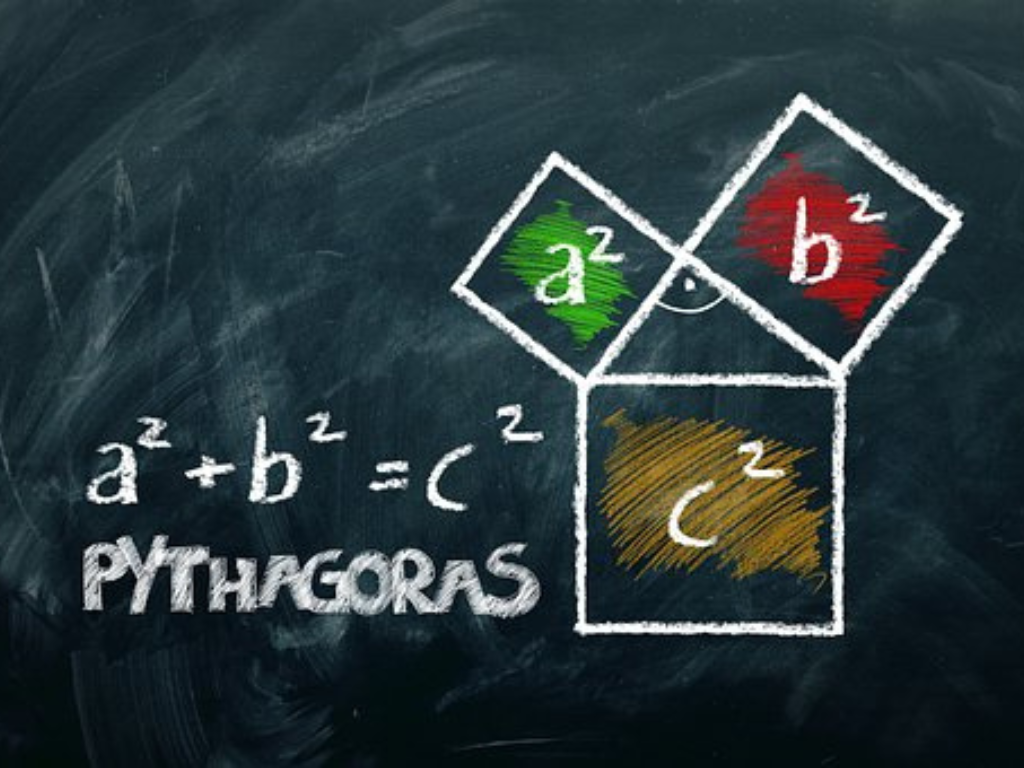## Pythagoras Theorem (Boudhayana Theorem) Class 10th

Introduction The Pythagoras theorem is given by the Greek mathematician Pythagoras. Before Pythagoras, this theorem was discovered by Indian mathematician Boudhayana so this theorem is also known as Boudhayana Theorem. This theorem is also called Pythagorean Theorem. Statement – According to this theorem, In a right-angled triangle, the square of the hypotenuse side is equal …